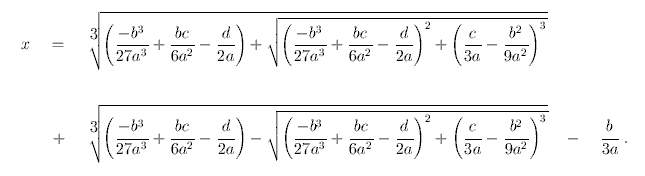# Finding X Intercepts

## Homework Statement

Find x intercept of:

x^3-3 x^2+3

## Homework Equations

I can clearly see three x intercepts on the graph but can not determine them mathematically. Quadratic formula and calculator give non real answer. Book says: ( -.879, 0) (1.347,0) (2.5232, 0)

## The Attempt at a Solution

I am totally stuck as the quadratic gives a negative discriminant and a non real answer.... yet there are indeed three intercepts.

What am I doing wrong?

Thanks,

Warren

I don't think you could use the quad. formula here because it's not in the form of ax^2+bx+c. Did you go over division? I believe you have to use long division to find the zeroes.

I don't think you could use the quad. formula here because it's not in the form of ax^2+bx+c.
I agree.
Well, I don't know about finding exact roots, but you can get a general idea of where the zeroes are by plugging in a few numbers. For example, with x=1 you get a positive answer, but with x=-1 you get a negative answer. So in between x=1 and x=-1 there is a root.

I don't think you could use the quad. formula here because it's not in the form of ax^2+bx+c. Did you go over division? I believe you have to use long division to find the zeroes.

D'oh! Of course! Man, it is possible to study too long...! I'm still at a loss...

I'm not sure how to work the division...any hints?....

Thanks,

Warren

eumyang
Homework Helper
There's the Rational Roots Theorem, but it's not going to work in this case, because you can see that all three roots are irrational. There's the Cubic Formula, but it's very complicated to use. Outside of those two methods, you're not going to be able to solve this algebraically.

Durand Kerner method?

So....

Use the calculator and zero function to estimate is all that could possibly be expected from a calc 1 student right?
I gotta say this is so typical of the Larson Calculus of a Single Variable book! Each chapter has problems that are near impossible to solve with the instruction given to that point.

When learning the product rule several problems were given that demanded quotient rule and the answer book gave the the answer as quotient rule form. Unfortunatly quotient rule isn't presented till the next section!

Thanks,

Warren

I have a friend who took college algebra for the extra A (he tested out of it) and he swears they had to solve cubic equations.

But... here you go.